# Yr7-Sequences.pptx

3 de Aug de 2022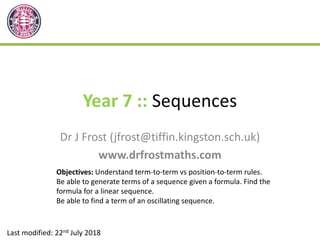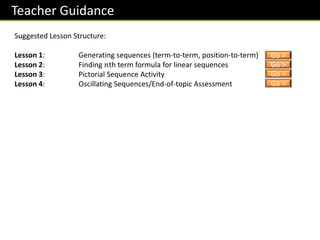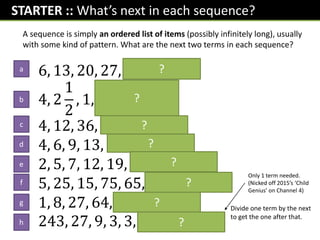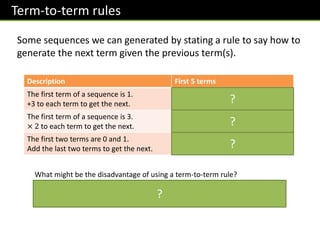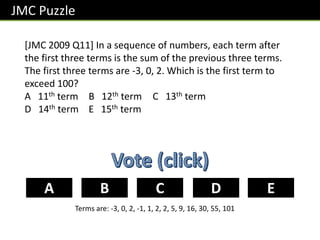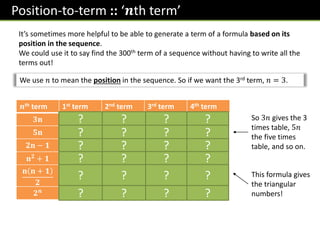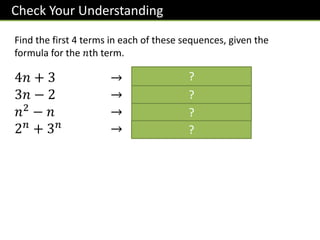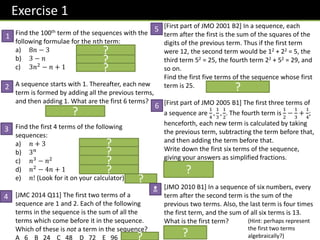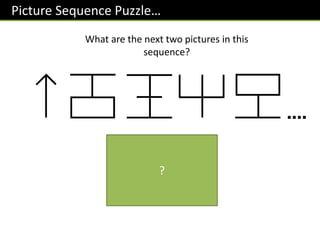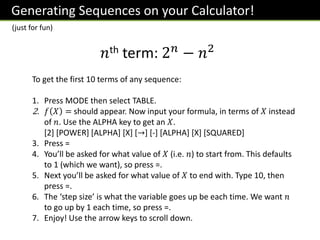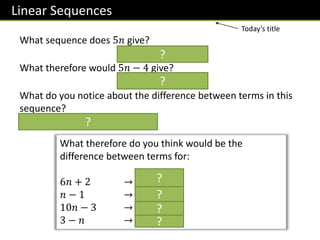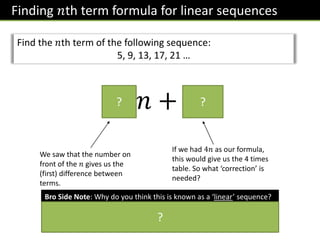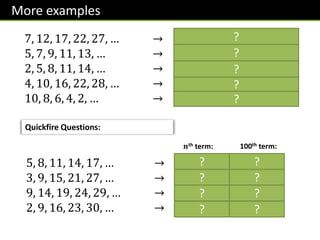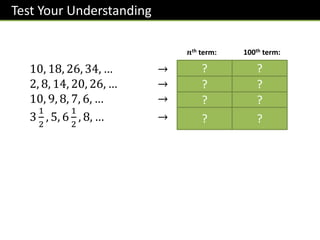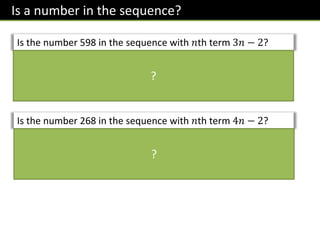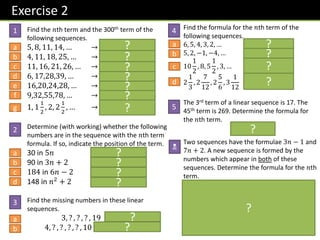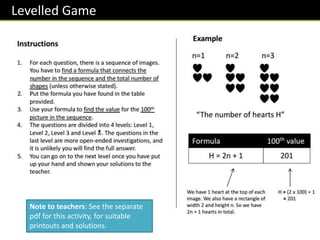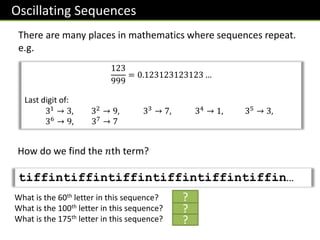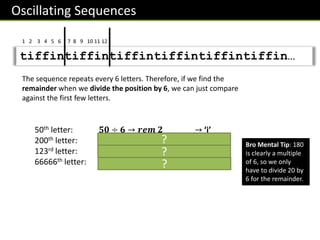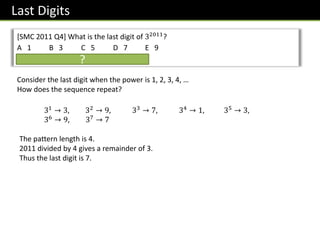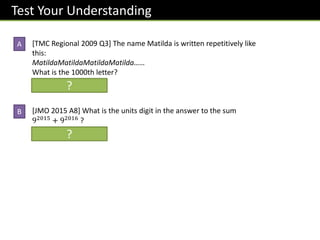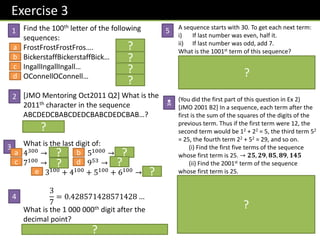1 de 25

### Yr7-Sequences.pptx

• 1. Year 7 :: Sequences Dr J Frost (jfrost@tiffin.kingston.sch.uk) www.drfrostmaths.com Last modified: 22nd July 2018 Objectives: Understand term-to-term vs position-to-term rules. Be able to generate terms of a sequence given a formula. Find the formula for a linear sequence. Be able to find a term of an oscillating sequence.
• 2. www.drfrostmaths.com Everything is completely free. Why not register? Teaching videos with topic tests to check understanding. Register now to interactively practise questions on this topic, including past paper questions and extension questions (including UKMT). Teachers: you can create student accounts (or students can register themselves), to set work, monitor progress and even create worksheets. Questions organised by topic, difficulty and past paper. Dashboard with points, trophies, notifications and student progress. With questions by:
• 3. Teacher Guidance Suggested Lesson Structure: Lesson 1: Generating sequences (term-to-term, position-to-term) Lesson 2: Finding 𝑛th term formula for linear sequences Lesson 3: Pictorial Sequence Activity Lesson 4: Oscillating Sequences/End-of-topic Assessment Go > Go > Go > Go >
• 4. STARTER :: What’s next in each sequence? 6, 13, 20, 27, 𝟑𝟒, 𝟒𝟏, … 4, 2 1 2 , 1, − 𝟏 𝟐 , −𝟐, … 4, 12, 36, 𝟏𝟎𝟖, 𝟑𝟐𝟒, … 4, 6, 9, 13, 𝟏𝟖, 𝟐𝟒, … 2, 5, 7, 12, 19, 𝟑𝟏, 𝟓𝟎, … 5, 25, 15, 75, 65, 𝟑𝟐𝟓, 𝟑𝟏𝟓, … 1, 8, 27, 64, 𝟏𝟐𝟓, 𝟐𝟏𝟔, … 243, 27, 9, 3, 3, 𝟏, … A sequence is simply an ordered list of items (possibly infinitely long), usually with some kind of pattern. What are the next two terms in each sequence? ? ? ? ? ? ? ? ? Only 1 term needed. (Nicked off 2015’s ‘Child Genius’ on Channel 4) Divide one term by the next to get the one after that. a b c d e f g h
• 5. Term-to-term rules Some sequences we can generated by stating a rule to say how to generate the next term given the previous term(s). Description First 5 terms The first term of a sequence is 1. +3 to each term to get the next. 1, 4, 7, 10, 13 The first term of a sequence is 3. × 2 to each term to get the next. 3, 6, 12, 24, 48 The first two terms are 0 and 1. Add the last two terms to get the next. 0, 1, 1, 2, 3 (known as the Fibonacci sequence) ? ? ? What might be the disadvantage of using a term-to-term rule? To get a particular term in the sequence, we have to generate all the terms in the sequence before it. This is rather slow if you say want to know the 1000th term! ?
• 6. [JMC 2009 Q11] In a sequence of numbers, each term after the first three terms is the sum of the previous three terms. The first three terms are -3, 0, 2. Which is the first term to exceed 100? A 11th term B 12th term C 13th term D 14th term E 15th term JMC Puzzle C B A D E Terms are: -3, 0, 2, -1, 1, 2, 2, 5, 9, 16, 30, 55, 101
• 7. Position-to-term :: ‘𝒏th term’ It’s sometimes more helpful to be able to generate a term of a formula based on its position in the sequence. We could use it to say find the 300th term of a sequence without having to write all the terms out! We use 𝑛 to mean the position in the sequence. So if we want the 3rd term, 𝑛 = 3. 𝒏th term 1st term 2nd term 3rd term 4th term 𝟑𝐧 3 6 9 12 𝟓𝐧 5 10 15 20 𝟐𝐧 − 𝟏 1 3 5 7 𝐧𝟐 + 𝟏 2 5 10 17 𝐧 𝐧 + 𝟏 𝟐 1 3 6 10 𝟐𝒏 2 4 8 16 This formula gives the triangular numbers! So 3𝑛 gives the 3 times table, 5𝑛 the five times table, and so on. ? ? ? ? ? ? ? ? ? ? ? ? ? ? ? ? ? ? ? ? ? ? ? ?
• 8. Check Your Understanding Find the first 4 terms in each of these sequences, given the formula for the 𝑛th term. 4𝑛 + 3 → 𝟕, 𝟏𝟏, 𝟏𝟓, 𝟏𝟗 3𝑛 − 2 → 𝟏, 𝟒, 𝟕, 𝟏𝟎 𝑛2 − 𝑛 → 𝟎, 𝟐, 𝟔, 𝟏𝟐 2𝑛 + 3𝑛 → 𝟓, 𝟏𝟑, 𝟑𝟓, 𝟗𝟕 ? ? ? ?
• 9. Challenge Your Neighbour Create your own 𝑛th term formula and find the first four items of your sequence. (Find something interesting without it being too overly tedious to calculate) Once done, write the formula in the BACK of your neighbour’s book (your neighbour will do likewise in your book). You then need to work out the first four terms of their sequence. Swap back and check they got it right.
• 10. Exercise 1 [First part of JMO 2001 B2] In a sequence, each term after the first is the sum of the squares of the digits of the previous term. Thus if the first term were 12, the second term would be 12 + 22 = 5, the third term 52 = 25, the fourth term 22 + 52 = 29, and so on. Find the first five terms of the sequence whose first term is 25. 25, 29, 85, 89, 145 [First part of JMO 2005 B1] The first three terms of a sequence are 1 4 , 1 3 , 1 2 . The fourth term is 1 2 − 1 3 + 1 4 ; henceforth, each new term is calculated by taking the previous term, subtracting the term before that, and then adding the term before that. Write down the first six terms of the sequence, giving your answers as simplified fractions. 𝟏 𝟒 , 𝟏 𝟑 , 𝟏 𝟐 , 𝟓 𝟏𝟐 , 𝟏 𝟒 , 𝟏 𝟑 [JMO 2010 B1] In a sequence of six numbers, every term after the second term is the sum of the previous two terms. Also, the last term is four times the first term, and the sum of all six terms is 13. What is the first term? Solution: 𝟏 𝟏 𝟒 Find the 100th term of the sequences with the following formulae for the 𝑛th term: a) 8𝑛 − 3 797 b) 3 − 𝑛 -97 c) 3𝑛2 − 𝑛 + 1 29901 A sequence starts with 1. Thereafter, each new term is formed by adding all the previous terms, and then adding 1. What are the first 6 terms? 1, 2, 4, 8, 16, 32 Find the first 4 terms of the following sequences: a) 𝑛 + 3 4, 5, 6, 7 b) 3𝑛 3, 9, 27, 81 c) 𝑛3 − 𝑛2 0, 4, 18, 48 d) 𝑛2 − 4𝑛 + 1 -2, -3, -2, 1 e) 𝑛! (Look for it on your calculator) 1, 2, 6, 24 [JMC 2014 Q11] The first two terms of a sequence are 1 and 2. Each of the following terms in the sequence is the sum of all the terms which come before it in the sequence. Which of these is not a term in the sequence? A 6 B 24 C 48 D 72 E 96 Solution: D (Hint: perhaps represent the first two terms algebraically?) ? ? ? ? ? ? ? ? ? ? ? ? 1 2 3 4 5 6 N
• 11. Picture Sequence Puzzle… What are the next two pictures in this sequence? It’s the numbers 1, 2, 3, … but reflected. Sneaky! ?
• 12. Generating Sequences on your Calculator! 𝑛th term: 2𝑛 − 𝑛2 To get the first 10 terms of any sequence: 1. Press MODE then select TABLE. 2. 𝑓 𝑋 = should appear. Now input your formula, in terms of 𝑋 instead of 𝑛. Use the ALPHA key to get an 𝑋.  [POWER] [ALPHA] [X] [→] [-] [ALPHA] [X] [SQUARED] 3. Press = 4. You’ll be asked for what value of 𝑋 (i.e. 𝑛) to start from. This defaults to 1 (which we want), so press =. 5. Next you’ll be asked for what value of 𝑋 to end with. Type 10, then press =. 6. The ‘step size’ is what the variable goes up be each time. We want 𝑛 to go up by 1 each time, so press =. 7. Enjoy! Use the arrow keys to scroll down. (just for fun)
• 13. Linear Sequences What sequence does 5𝑛 give? 𝟓, 𝟏𝟎, 𝟏𝟓, 𝟐𝟎, … What therefore would 5𝑛 − 4 give? 𝟏, 𝟔, 𝟏𝟏, 𝟏𝟔, … What do you notice about the difference between terms in this sequence? It goes up by 5 each time. What therefore do you think would be the difference between terms for: 6𝑛 + 2 → 6 𝑛 − 1 → 1 10𝑛 − 3 → 10 3 − 𝑛 → −1 ? ? ? ? ? ? ? Today’s title
• 14. Finding 𝑛th term formula for linear sequences Find the 𝑛th term of the following sequence: 5, 9, 13, 17, 21 … 4𝑛 + 1 ? ? We saw that the number on front of the 𝑛 gives us the (first) difference between terms. If we had 4𝑛 as our formula, this would give us the 4 times table. So what ‘correction’ is needed? Bro Side Note: Why do you think this is known as a ‘linear’ sequence? If you plotted each position with the term on some axes (e.g. for this sequence (1,5),(2,9),(3,13),(4,17), …, it would form a straight line. The word ‘linear’ means ‘straight’. ?
• 15. More examples 7, 12, 17, 22, 27, … → 𝟓𝒏 + 𝟐 5, 7, 9, 11, 13, … → 𝟐𝒏 + 𝟑 2, 5, 8, 11, 14, … → 𝟑𝒏 − 𝟏 4, 10, 16, 22, 28, … → 𝟔𝒏 − 𝟐 10, 8, 6, 4, 2, … → −𝟐𝒏 + 𝟏𝟐 (or 𝟏𝟐 − 𝟐𝒏) Quickfire Questions: 5, 8, 11, 14, 17, … → 3𝑛 + 2 3, 9, 15, 21, 27, … → 6𝑛 − 3 9, 14, 19, 24, 29, … → 5𝑛 + 4 2, 9, 16, 23, 30, … → 7𝑛 − 5 100th term: 𝒏th term: 302 597 504 695 ? ? ? ? ? ? ? ? ? ? ? ? ?
• 16. Test Your Understanding 10, 18, 26, 34, … → 8𝑛 + 2 2, 8, 14, 20, 26, … → 6𝑛 − 4 10, 9, 8, 7, 6, … → 11 − 𝑛 3 1 2 , 5, 6 1 2 , 8, … → 3 2 𝑛 + 2 100th term: 𝒏th term: 802 596 −89 152 ? ? ? ? ? ? ? ?
• 17. Is a number in the sequence? Is the number 598 in the sequence with 𝑛th term 3𝑛 − 2? Could we obtain 598 using the 𝟑𝒏 − 𝟐 formula? Yes! Working backwards, we see 𝒏 = 𝟐𝟎𝟎. So 598 is the 200th term in the sequence. Is the number 268 in the sequence with 𝑛th term 4𝑛 − 2? No. 𝟒𝒏 − 𝟐 = 𝟐𝟔𝟖 But adding 2 we get 270, and 270 is not divisible by 4. ? ?
• 18. Exercise 2 Find the 𝑛th term and the 300th term of the following sequences. 5, 8, 11, 14, … → 𝟑𝒏 + 𝟐, 𝟗𝟎𝟐 4, 11, 18, 25, … → 𝟕𝒏 − 𝟑, 𝟐𝟎𝟗𝟕 11, 16, 21, 26, … → 𝟓𝒏 + 𝟔, 𝟏𝟓𝟎𝟔 6, 17,28,39, … → 𝟏𝟏𝒏 − 𝟓, 𝟑𝟐𝟗𝟓 16,20,24,28, … → 𝟒𝒏 + 𝟏𝟐, 𝟏𝟐𝟏𝟐 9,32,55,78, … → 𝟐𝟑𝒏 − 𝟏𝟒, 𝟔𝟖𝟖𝟔 1, 1 1 2 , 2, 2 1 2 , … → 𝟏 𝟐 𝒏 + 𝟏 𝟐 , 𝟏𝟓𝟎 𝟏 𝟐 Determine (with working) whether the following numbers are in the sequence with the 𝑛th term formula. If so, indicate the position of the term. 30 in 5𝑛 Yes (6th term) 90 in 3𝑛 + 2 No 184 in 6𝑛 − 2 Yes (31st term) 148 in 𝑛2 + 2 No Find the missing numbers in these linear sequences. 3, ? , ? , ? , 19 𝟕, 𝟏𝟏, 𝟏𝟓 4, ? , ? , ? , ? , 10 (𝟓. 𝟐, 𝟔. 𝟒, 𝟕. 𝟔, 𝟖. 𝟖) Find the formula for the 𝑛th term of the following sequences. 6, 5, 4, 3, 2, … 𝟕 − 𝒏 5, 2, −1, −4, … 𝟖 − 𝟑𝒏 10 1 2 , 8, 5 1 2 , 3, … 𝟏𝟑 − 𝟓 𝟐 𝒏 2 1 3 , 2 7 12 , 2 5 6 , 3 1 12 𝟏 𝟒 𝒏 + 𝟐𝟓 𝟏𝟐 The 3rd term of a linear sequence is 17. The 45th term is 269. Determine the formula for the 𝑛th term. 𝟔𝒏 − 𝟏 Two sequences have the formulae 3𝑛 − 1 and 7𝑛 + 2. A new sequence is formed by the numbers which appear in both of these sequences. Determine the formula for the 𝑛th term. 𝟐𝟏𝒏 + 𝟐 Whatever the first number is that coincides, we’ll see it 21 later because this is the ‘lowest common multiple’ of 3 and 7. Thus we know the formula is of the form 𝟐𝟏𝒏 + □. It’s then simply a case of identifying which number this is (2). This principle is known as the ‘Chinese Remainder Theorem’. 2 1 3 4 5 N a b c d e f g a b c d a b a b c d ? ? ? ? ? ? ? ? ? ? ? ? ? ? ? ? ? ? ?
• 19. Levelled Game Note to teachers: See the separate pdf for this activity, for suitable printouts and solutions.
• 20. Oscillating Sequences There are many places in mathematics where sequences repeat. e.g. 123 999 = 0.123123123123 … Last digit of: 31 → 3, 32 → 9, 33 → 7, 34 → 1, 35 → 3, 36 → 9, 37 → 7 How do we find the 𝑛th term? tiffintiffintiffintiffintiffintiffin… What is the 60th letter in this sequence? ‘n’ What is the 100th letter in this sequence? ‘f’ What is the 175th letter in this sequence? ‘t’ ? ? ?
• 21. Oscillating Sequences tiffintiffintiffintiffintiffintiffin… 1 2 3 4 5 6 7 8 9 10 11 12 The sequence repeats every 6 letters. Therefore, if we find the remainder when we divide the position by 6, we can just compare against the first few letters. 50th letter: 𝟓𝟎 ÷ 𝟔 → 𝒓𝒆𝒎 𝟐 → ‘i’ 200th letter: 𝟐𝟎𝟎 ÷ 𝟔 → 𝒓𝒆𝒎 𝟐 → ‘i’ 123rd letter: 𝟏𝟐𝟑 ÷ 𝟔 → 𝒓𝒆𝒎 𝟑 → ‘f’ 66666th letter: 𝟔𝟔𝟔𝟔𝟔 ÷ 𝟔 → 𝒓𝒆𝒎 𝟎 → ‘n’ ? ? ? Bro Mental Tip: 180 is clearly a multiple of 6, so we only have to divide 20 by 6 for the remainder.
• 22. Last Digits [SMC 2011 Q4] What is the last digit of 32011 ? A 1 B 3 C 5 D 7 E 9 Solution: D ? Consider the last digit when the power is 1, 2, 3, 4, … How does the sequence repeat? 31 → 3, 32 → 9, 33 → 7, 34 → 1, 35 → 3, 36 → 9, 37 → 7 The pattern length is 4. 2011 divided by 4 gives a remainder of 3. Thus the last digit is 7.
• 23. Test Your Understanding [TMC Regional 2009 Q3] The name Matilda is written repetitively like this: MatildaMatildaMatildaMatilda…… What is the 1000th letter? Solution: d [JMO 2015 A8] What is the units digit in the answer to the sum 92015 + 92016 ? Solution: 0 A B ? ?
• 24. Exercise 3 Find the 100th letter of the following sequences: FrostFrostFrostFros…. t BickerstaffBickerstaffBick… B IngallIngallIngall… a OConnellOConnell… n [JMO Mentoring Oct2011 Q2] What is the 2011th character in the sequence ABCDEDCBABCDEDCBABCDEDCBAB…? Solution: C What is the last digit of: 4300 → 𝟔 51000 → 𝟓 7100 → 𝟏 953 → 𝟗 3100 + 4100 + 5100 + 6100 → 𝟖 3 7 = 0.428571428571428 … What is the 1 000 000th digit after the decimal point? 𝟏𝟎𝟎𝟎𝟎𝟎𝟎 ÷ 𝟔 gives rem 4. So answer is 5. A sequence starts with 30. To get each next term: i) If last number was even, half it. ii) If last number was odd, add 7. What is the 1001st term of this sequence? 8th term to 11th term repeats 8, 4, 2, 1. 𝟏𝟎𝟎𝟏 ÷ 𝟖 gives remainder of 1. In the 8th-11th range this corresponds to the 9th term (1+4+4=9) which is 4. (You did the first part of this question in Ex 2) [JMO 2001 B2] In a sequence, each term after the first is the sum of the squares of the digits of the previous term. Thus if the first term were 12, the second term would be 12 + 22 = 5, the third term 52 = 25, the fourth term 22 + 52 = 29, and so on. (i) Find the first five terms of the sequence whose first term is 25. → 𝟐𝟓, 𝟐𝟗, 𝟖𝟓, 𝟖𝟗, 𝟏𝟒𝟓 (ii) Find the 2001st term of the sequence whose first term is 25. (ii) After, this sequence goes: 42, 20, 4, 16, 37, 58, 89, 145, …. We can see there’s a repeating sequence from the 4th term to the 11th term (that’s 8 terms long). 𝟐𝟎𝟎𝟏 ÷ 𝟖 gives a remainder of 1. But we don’t want the 1st term (which wasn’t in the repeating sequence) but the 1+8=9th term, which is 16. 1 2 3 4 5 N a b c d a b c d e ? ? ? ? ? ? ? ? ? ? ? ? ?